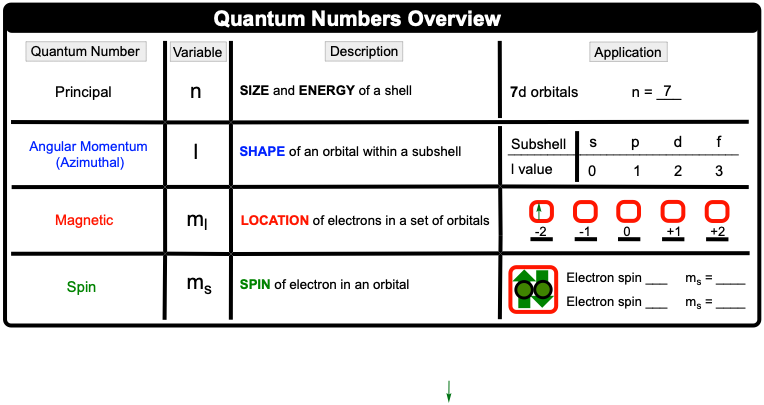Clutch Prep is now a part of Pearson
Ch.8 - Periodic Properties of the ElementsWorksheetSee all chapters

# The Electron Configuration: Quantum Numbers

See all sections
Sections
The Electron Configuration
The Electron Configuration: Condensed
The Electron Configurations: Exceptions
The Electron Configuration: Ions
Paramagnetism and Diamagnetism
The Electron Configuration: Quantum Numbers
Valence Electrons of Elements
Periodic Trend: Metallic Character
Periodic Trend: Ionization Energy
Periodic Trend: Successive Ionization Energies
Periodic Trend: Electron Affinity
Periodic Trend: Electronegativity
Periodic Trend: Effective Nuclear Charge
Periodic Trend: Cumulative

The Quantum Numbers ultimately can be used to determine the precise location of an electron.

###### Electron Configuration & Quantum Numbers

Concept #1: Counting Electrons

Example #1: Determine the number of the electron highlighted within the electron orbital diagram.

Concept #2: Quantum NumbersExample #2: Give the set of four quantum numbers for the 6th electron of the oxygen atom.

Practice: Give the set of four quantum numbers that represent the indicated electron in each of the following elements.

Mg (7th electron)

Practice: Give the set of four quantum numbers that represent the indicated electron in each of the following elements.

Cl (18th electron)

Practice: Give the set of four quantum numbers that represent the indicated electron in each of the following elements.

Cr3+ (21st electron)